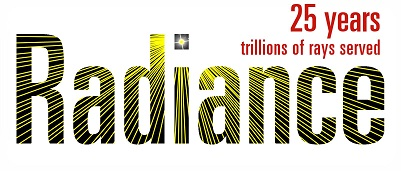# Merge the RGB components of dctimestep result (irradiance file)

Dear all,

I have created a daylight coefficients file with the command:

rfluxmtx -parameters oct_file.oct < pointsfile.pts > illumfile

Additionally, I have created a sky matrix with the folowing command:

gendaymtx -parameters weather.wea > sky

and in order to create the irradiance file I use the command:

dctimestep illumfile < sky > irrfile

to achieve the multiplication between the daylight coefficient matrix and the sky matrix.
First, I would like to ask, is this RGB multiplication implemented this way?:

Cr[i][j]=Ar[i]*Br[j]+Ar[i]*Br[j]+…+Ar[i][N]*Br[N][j]
Cg[i][j]=Ag[i]*Bg[j]+Ag[i]*Bg[j]+…+Ag[i][N]*Bg[N][j]
Cb[i][j]=Ab[i]*Bb[j]+Ab[i]*Bb[j]+…+Ab[i][N]*Bb[N][j]

Those 2 arrays contain 3 components of RGB in each element, so the irradiance file will contain 3 components of RGB in each element too.
In order to reduce the size of the result file irradiance, I merge the RGB numbers to one number with the formula (0.265*R + 0.67 G + 0.065B) at this command:
rmtxop -parameters 0.265 0.67 0.065 - < irrfile > result

Now, what I am trying to do is to merge the RGB contents of the illumfile and the skyvector before the multiplication.

But if I use the same formula (0.265*R + 0.67 G + 0.065B) to each element of the daylight coefficient and the sky vector and then I multiplicate them, I do not have the same result as previous.

Any ideas for how I can achieve the same result?
Because if I had 3 components just in the illumfile and 1 component in the sky matrix It would work.
Do I have to apply another formula for the both of the files?

Unless your values are all gray in either the sky file (which would be typical) or the daylight coefficient file (which is less usual), the precombination of RGB prior to multiplication will yield different results.

If you are just interested in saving time, you should use the binary versions of the files. Most of the time is spent converting from binary float to ASCII text and back, not the actual vector/matrix multiplication itself.

Dear Greg_Ward,